## 4.8 Segment Definition Primitives

Segment primitives can be used directly to construct shapes. A shape is constructed in this way by generating a number of segments, and adding these segments to the shape. Segment definition primitives are only meant for internal use in Bifrost. It is not recommended to use these primitives, except for defining circular spline segments, but instead to use the shape definition operations described in Shape Definition Primitives.

### 4.8.1 LineSegment

A line segment is described by two control points: Begin (= FirstPoint) and End (= LastPoint). A line segment is constructed by assigning values to the Begin and End points:

 ```aSeg:@LineSegment (# do (100,100) -> begin; (200,200) -> end; #)```Resulting line segment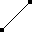### 4.8.2 SplineSegment

As mentioned earlier there are two different kinds of spline segments: circular and non-circular splines. A circular spline is always considered closed, and a non-circular spline is considered closed if the last operation performed is Close. Non-circular splines are typically used in construction of shapes consisting of both line and spline segments, and circular splines are useful for making circles and ellipses. Spline segments have three operations used for creating the spline:

Open.
Takes one point as argument. The point is the first control point of the spline, and the spline is prepared to be constructed with further control points using AddControl.
This operation just adds a specified control point to the spline.
Close.
Closes a non-circular spline by adding FirstPoint to the spline definition. The operation has no effect on a circular spline. Notice, that it is still possible to add further control points to the spline definition after the Close operation.

The following examples illustrate the use of AddControl and Close for a non-circular spline:

 ```aBow: @NonCircularSplineSegment (# do ( 0,100) -> Open; (100,100) -> AddControl; (100, 0) -> AddControl; ( 0, 0) -> AddControl; #);```Open non-circular spline: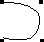```aDrop: @NonCircularSplineSegment (# do ( 0,100) -> Open; (100,100) -> AddControl; (100, 0) -> AddControl; ( 0, 0) -> AddControl; close; #);```Closed non-circular spline: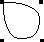The next examples illustrate the use of AddControl for circular splines:

 ```aSpline: @CircularSplineSegment (# do ( 0,100) -> Open; (100,100) -> AddControl; (100, 0) -> AddControl; #);```Circular spline with three controlpoints: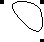```aCircle: @CircularSplineSegment (# do ( 0,100) -> Open; (100,100) -> AddControl; (100, 0) -> AddControl; ( 0, 0) -> AddControl; #);```Circular spline with four controlpoints: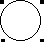Notice that a circular spline is inherently closed.

### 4.8.3 Adding Segments to Shapes

When the segment is defined it can be added to a shape with two shape primitives: AddLine and AddSpline.

Adds a line segment to the shape. In case where FirstPoint of the line segment does not coincide with LastPoint of the shape, the line segment is moved to the appropriate position.
AddSpline adds a spline to the shape in construction. In case where FirstPoint of the spline segment does not coincide with LastPoint of the shape, the spline segment is moved to the appropriate position.

The following example illustrates the use of AddLine:

 ```aLine: @LineSegment (# do (100,100) -> begin; (150,150) -> end; #); anotherLine: @LineSegment (# do (200,200) -> begin; (200,100) -> end; #); aShape: @Shape (# do aLine -> AddLine; anotherLine -> AddLine; #);```Two line segments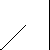Resulting shape: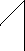The following example illustrates the use of and AddSpline using the two splines defined previously:

 ```aShape: @Shape (# do aCircle -> AddSpline; aDrop -> AddSpline; #);```Resulting shape: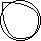In the case where the spline is not closed, i.e. FirstPoint does not coincide with LastPoint of the shape, the spline is moved to the appropriate position by AddSpline:

 ```aLine: @LineSegment (# do (100,100) -> begin; ( 50,150) -> end; #); anotherLine: @LineSegment (# do ( 50,150) -> begin; (150,200) -> end; #);```Two lines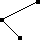```aSpline: @NonCircularSplineSegment (# do ( 0,200) -> Open; ( 50,250) -> AddControl; (100,200) -> AddControl; (100,100) -> AddControl; ( 50, 50) -> AddControl; #);```A Spline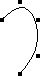```aShape: @Shape (# do aLine -> AddLine; anotherLine -> AddLine; aSpline -> AddSpline; #);```Resulting shape: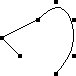It should be clear from the examples that it is complicated to use the segment definition primitives for shape construction. Therefore, for purpose of convenience and to make the graphics system more powerful, Bifrost also includes the small shape construction language presented earlier.

 The Bifrost Graphics System - Reference Manual © 1991-2004 Mjølner Informatics [Modified: Tuesday October 24th 2000 at 15:02]